Worksheets and No Prep Teaching Resources
Math Worksheets

# Counting and Number Practice Worksheets

If students can't count, they can't learn math, so establishing this important skill in a fun, lively way paves the way for a great attitude toward math that will build future success for young mathematicians. Great graphics and a systematic approach to counting through 99 make these worksheets a winner for every student.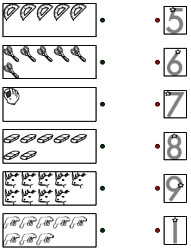Counting to Nine Math Workbook with Fidget Spinner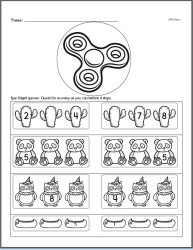Counting to 9 Workbook
Counting to Nineteen Math Workbook with Fidget Spinner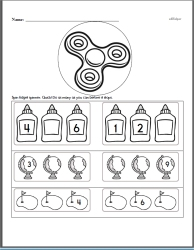Counting to 19 Workbook
Counting to Ninety-nine Math Workbook with Fidget Spinner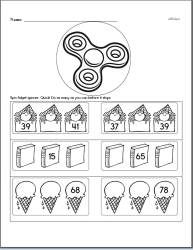Counting to 99 Workbook

Matching: Number of Images and the Number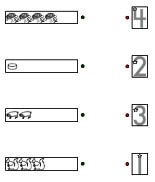Matching: Counting numbers 1 to 4 Matching: Counting numbers 5 to 9 Matching: Counting numbers 1 to 9 Matching: Counting numbers 10 to 15

Counting Chart for Bulletin Board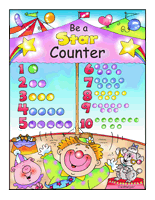"Be a Star Counter" Basics Chart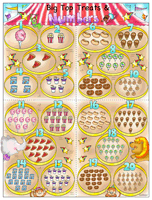"Big Top Treats & Numbers" Jumbo Chart

Count and Circle Number - Complete Number Chart Given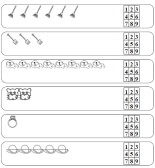Counting from 1 to 5 Counting from 1 to 9 Counting from 6 to 9 Counting from 1 to 12 Counting from 9 to 12 Counting from 1 to 15 Counting from 9 to 15

Count and Circle Number - Three Number Choices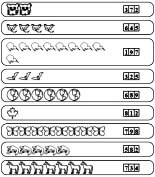Counting from 1 to 5 Counting from 1 to 9 Counting from 6 to 9 Counting from 1 to 12 Counting from 9 to 12 Counting from 1 to 15 Counting from 9 to 15

Cut and Paste - Counting and Numbers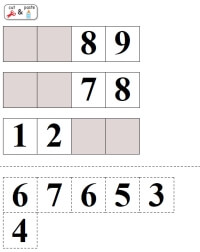Cut and Paste Counting - Numbers 1 to 9 (6 numbers and 1 missing number per row) Cut and Paste Counting - Numbers 1 to 9 (6 numbers and 2 missing numbers per row) Cut and Paste Counting - Numbers 1 to 9 (4 numbers and 1 missing number per row) Cut and Paste Counting - Numbers 1 to 9 (4 numbers and 2 missing numbers per row)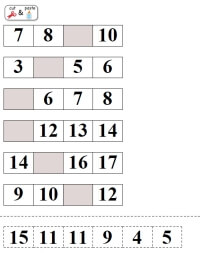Cut and Paste Counting - Numbers 1 to 19 (6 numbers and 1 missing number per row) Cut and Paste Counting - Numbers 1 to 19 (6 numbers and 2 missing numbers per row) Cut and Paste Counting - Numbers 1 to 19 (4 numbers and 1 missing number per row) Cut and Paste Counting - Numbers 1 to 19 (4 numbers and 2 missing numbers per row)

Counting Letters and Practice Writing a Letter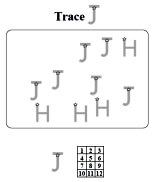Practice Writing and Counting Uppercase Letters Practice Writing and Counting Lowercase Letters

Counting Letters and Practice Writing Letters - Matching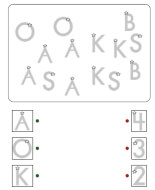Practice Writing and Counting Uppercase Letters with Matching Practice Writing and Counting Lowercase Letters with Matching

Drawing Pictures to Match Number (such as drawing four gumballs if "4" given)

Counting Activity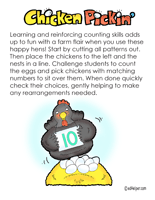Chicken Pickin'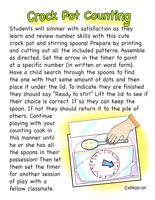Crock Pot Counting

Count and Color Mat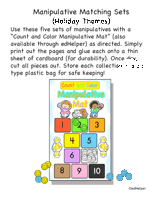Manipulative Matching Sets (Holiday Themes): use with count and color manipulative mat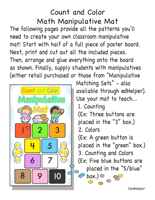"Count and Color Manipulative Mat"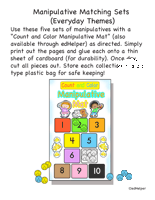"Matching Manipulative Sets" (Everyday Themes)

Folding Fingers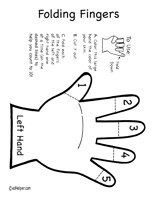Folding Fingers

Counting - Height and Length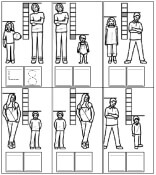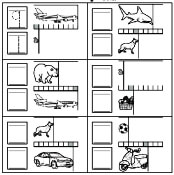Counting Shapes and Matching Totals
Total of 6 shapes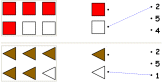Count two different colors (one answer is given)      Count two different colors

Total of 9 shapes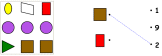Count two different colors (one answer is given)      Count two different colors      Two different shapes (one answer is given)      Two different shapes      Three different shapes (one answer is given)      Three different shapes      4-6 different shapes (one answer is given)      4-6 different shapes      3-6 different shapes with some of same shape but different colors (one answer is given)      3-6 different shapes with some of same shape but different colors

Total of 9 shapes - Similar shapes are not together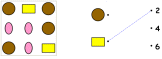Count two different colors (one answer is given)      Count two different colors      2-3 different shapes (one answer is given)      2-3 different shapes

Counting Shapes and Coloring
Total is from 4 to 8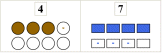Shapes to color have color spot      Only first problem has color spots      No color spots      No shapes are colored - gives total and color to use

Total is from 5 to 14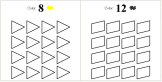Shapes to color have color spot      Only first problem has color spots      No color spots      No shapes are colored - gives total and color to use

Total is from 3 to 16 (smaller shapes and more problems per page)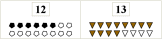Shapes to color have color spot      Only first problem has color spots      No color spots      No shapes are colored - gives total and color to use

Writing Number of Shapes and Addition of Shapes
Total is from 3 to 9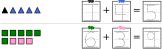Counting shapes and total both have trace numbers      One trace number given; other trace number and total are blank      All numbers blank

Total is from 5 to 9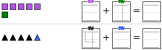Counting shapes and total both have trace numbers      One trace number given; other trace number and total are blank      All numbers blank

Total is from 10 to 15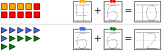Counting shapes and total both have trace numbers      One trace number given; other trace number and total are blank      All numbers blank

Total is from 10 to 20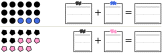Counting shapes and total both have trace numbers      One trace number given; other trace number and total are blank      All numbers blank

Counting and Coloring Shapes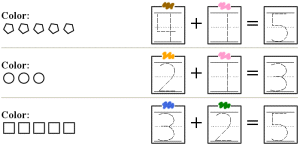Total is from 3 to 5      Numbers and total are trace numbers - color shapes      Only numbers given - color and write total Total is from 6 to 10      Numbers and total are trace numbers - color shapes      Only numbers given - color and write total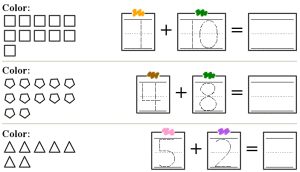Total is from 6 to 15      Numbers and total are trace numbers - color shapes      Only numbers given - color and write total Total is from 10 to 20      Numbers and total are trace numbers - color shapes      Only numbers given - color and write total

Counting Cars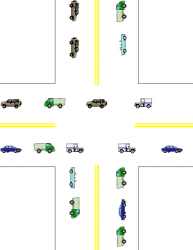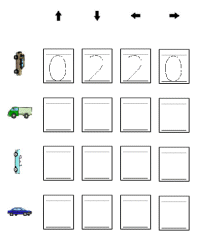Counting Food Items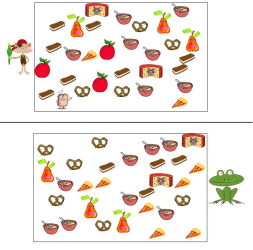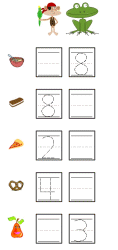Counting Colors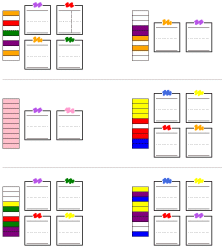Colors Are Together One Color Two Colors Three Colors Four Colors Mix of One to Four Colors Colors Are Mixed Up Two Colors Three Colors Four Colors Mix of One to Four Colors

Subtraction and Introduction to Subtraction

Odd and Even Numbers

Counting Money

Preschool Activity Books - New One Each Time!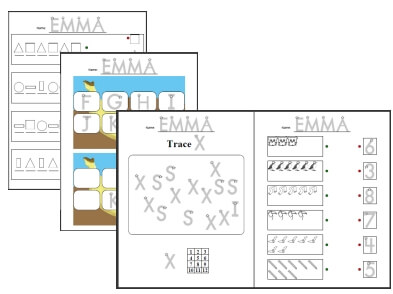Make a mixed preschool activity book (customize your book according to age and abilities)

Number Lines

Count and Compare Activity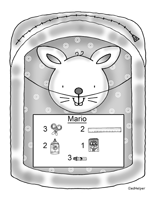Plan and Pack (Count and Compare)

A Counting Activity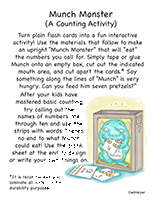Munch Monster

Comparing Numbers

Have a suggestion or would like to leave feedback?